Examples

Chapter 11 Class 12 Three Dimensional Geometry
Serial order wise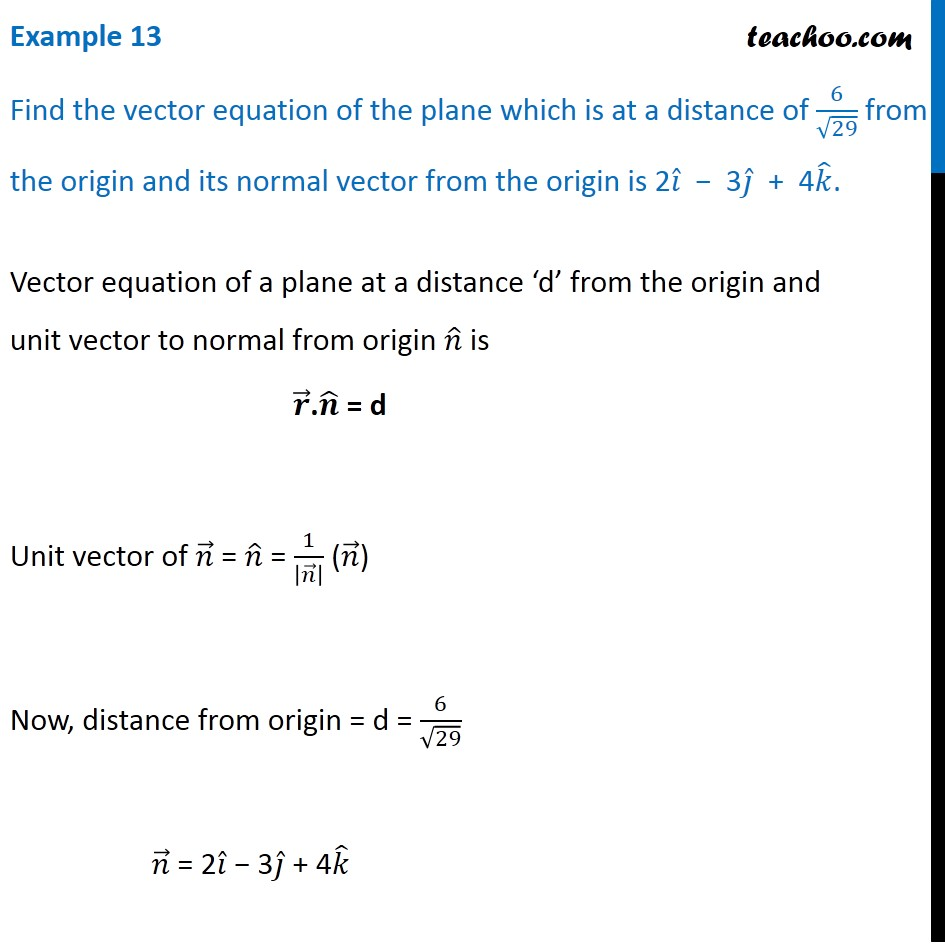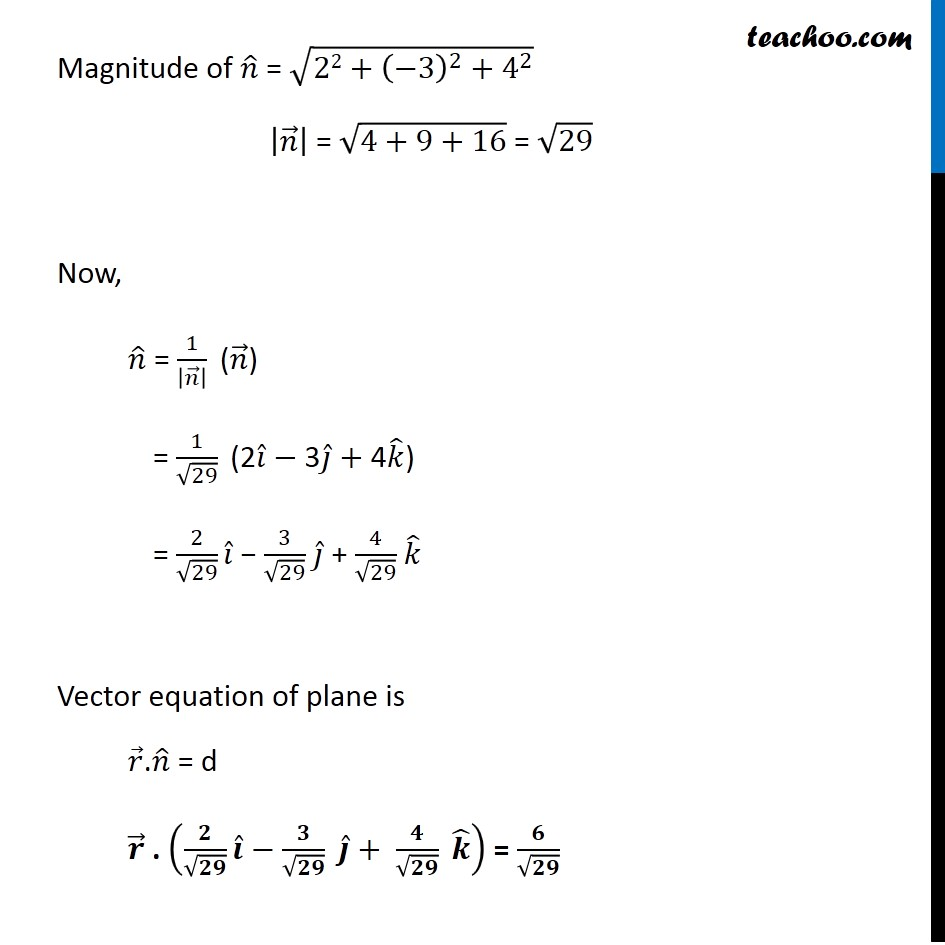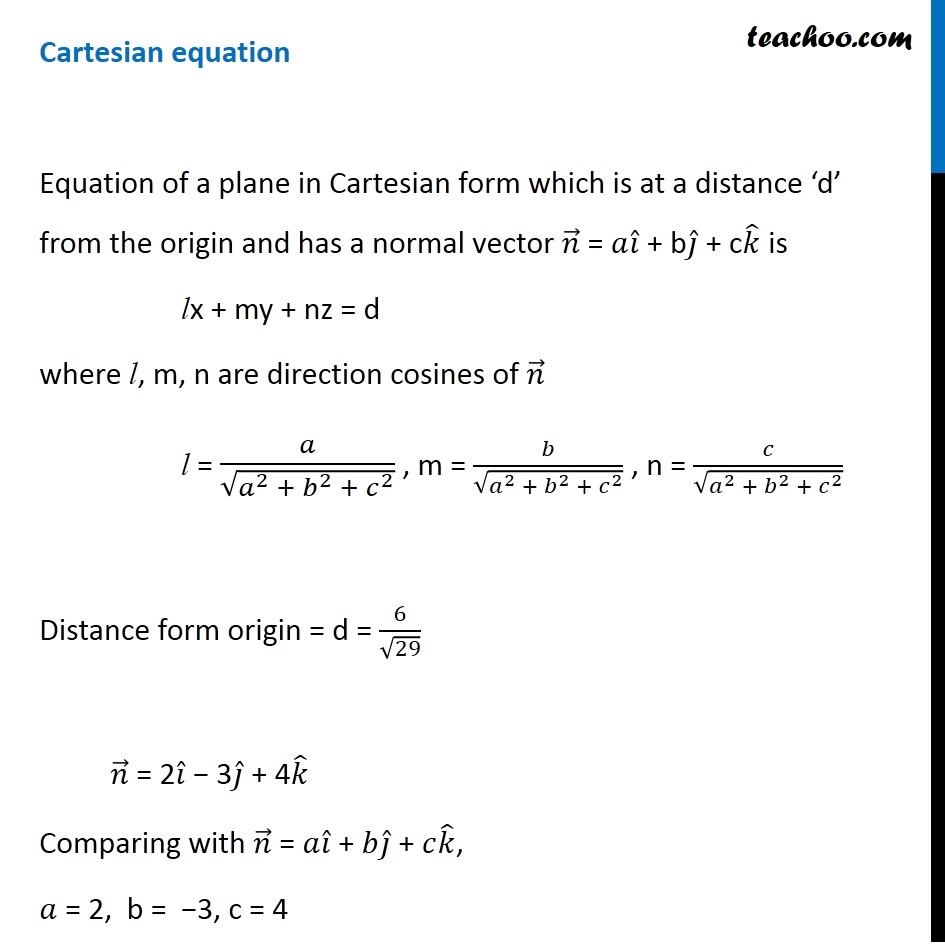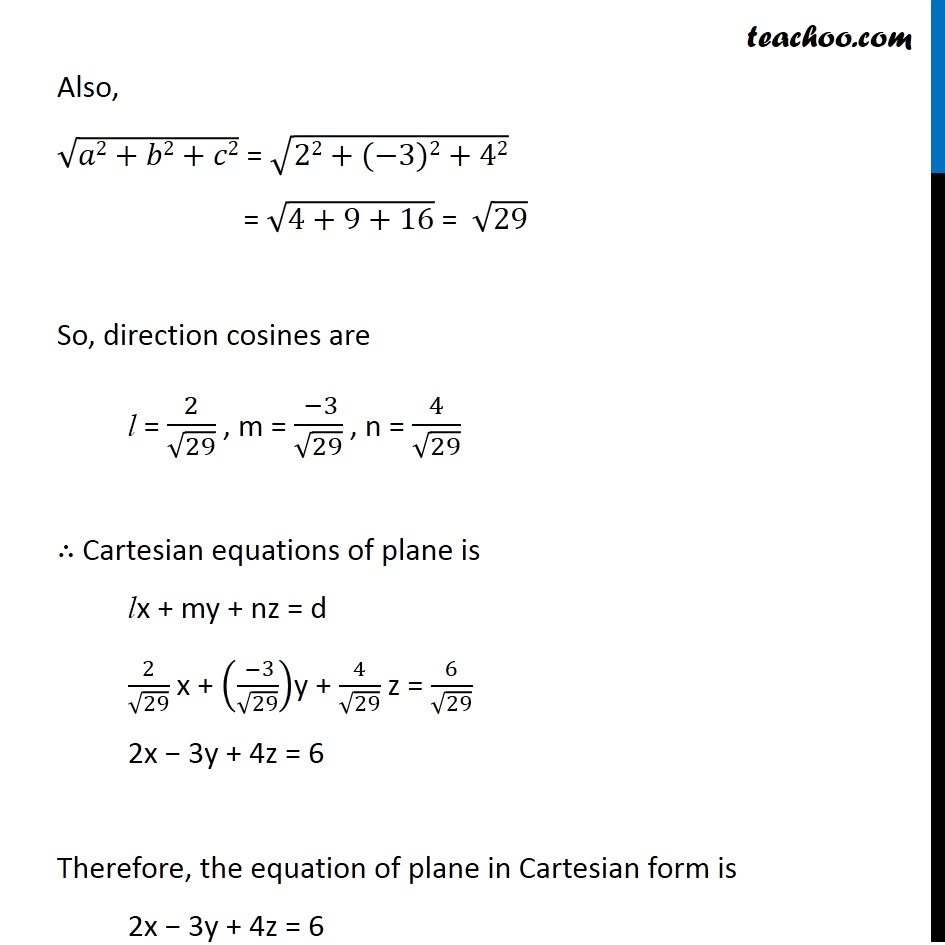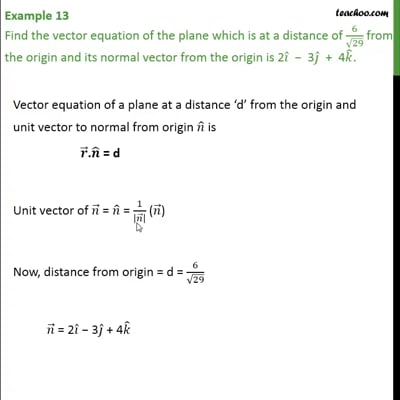This video is only available for Teachoo black users

Introducing your new favourite teacher - Teachoo Black, at only ₹83 per month

### Transcript

Example 13 Find the vector equation of the plane which is at a distance of 6/√29 from the origin and its normal vector from the origin is 2𝑖 ̂ − 3𝑗 ̂ + 4𝑘 ̂.Vector equation of a plane at a distance ‘d’ from the origin and unit vector to normal from origin 𝑛 ̂ is 𝒓 ⃗.𝒏 ̂ = d Unit vector of 𝑛 ⃗ = 𝑛 ̂ = 1/|𝑛 ⃗ | (𝑛 ⃗) Now, distance from origin = d = 6/√29 𝑛 ⃗ = 2𝑖 ̂ − 3𝑗 ̂ + 4𝑘 ̂ Magnitude of 𝑛 ̂ = √(22+(−3)^2+4^2 ) |𝑛 ⃗ | = √(4+9+16) = √29 Now, 𝑛 ̂ = 1/|𝑛 ⃗ | " (" 𝑛 ⃗")" = 1/√29 " (2" 𝑖 ̂−"3" 𝑗 ̂+"4" 𝑘 ̂")" = 2/√29 𝑖 ̂ − 3/√29 𝑗 ̂ + 4/√29 𝑘 ̂ Vector equation of plane is 𝑟 ⃗.𝑛 ̂ = d 𝒓 ⃗ . (𝟐/√𝟐𝟗 𝒊 ̂−𝟑/√𝟐𝟗 " " 𝒋 ̂+ 𝟒/√𝟐𝟗 " " 𝒌 ̂ ) = 𝟔/√𝟐𝟗 Cartesian equation Equation of a plane in Cartesian form which is at a distance ‘d’ from the origin and has a normal vector 𝑛 ⃗ = 𝑎𝑖 ̂ + b𝑗 ̂ + c𝑘 ̂ is lx + my + nz = d where l, m, n are direction cosines of 𝑛 ⃗ l = 𝑎/√(𝑎^2 + 𝑏^2 + 𝑐^2 ) , m = 𝑏/√(𝑎^2 + 𝑏^2 + 𝑐^2 ) , n = 𝑐/√(𝑎^2 + 𝑏^2 + 𝑐^2 ) Distance form origin = d = 6/√29 𝑛 ⃗ = 2𝑖 ̂ − 3𝑗 ̂ + 4𝑘 ̂ Comparing with 𝑛 ⃗ = 𝑎𝑖 ̂ + 𝑏𝑗 ̂ + 𝑐𝑘 ̂, 𝑎 = 2, b = −3, c = 4 Also, √(𝑎2+𝑏2+𝑐2) = √(22+(−3)2+42) = √(4+9+16) = √29 So, direction cosines are l = 2/√29 , m = ( −3)/√29 , n = 4/√29 ∴ Cartesian equations of plane is lx + my + nz = d 2/√29 x + (( −3)/√29)y + 4/√29 z = 6/√29 2x − 3y + 4z = 6 Therefore, the equation of plane in Cartesian form is 2x − 3y + 4z = 6